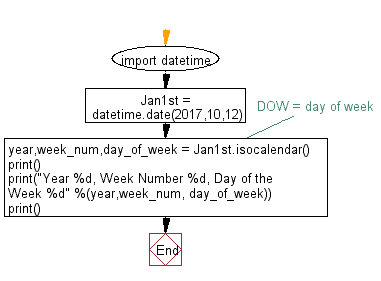﻿ Python: Get the current week - w3resource

# Python: Get the current week

## Python Datetime: Exercise-45 with Solution

Write a Python program to get the current week.

Sample Solution:-

Python Code:

``````import datetime
Jan1st = datetime.date(2017,10,12)
year,week_num,day_of_week = Jan1st.isocalendar() # DOW = day of week
print()
print("Year %d, Week Number %d, Day of the Week %d" %(year,week_num, day_of_week))
print()
```
```

Sample Output:

```Year 2017, Week Number 41, Day of the Week 4
```

Flowchart:Python Code Editor: